Definitions of Square Dance Calls and Concepts
Triple {formation}s Working {direction} Concept [C3B]

Triple formations Working direction is a precise way to state how to do some 12-Matrix calls. EN: 10
Triple formations Working direction \$B\$O(B, \$B\$\$\$/\$D\$+\$N(B 12-Matrix \$B%3!<%k\$r\$I\$N\$h\$&\$K9T\$&\$+\$K\$D\$\$\$F(B, \$B@53N\$K;X<(\$9\$k\$?\$a\$NJ}K!\$G\$9(B. JP: 10

This concept allows for 8-dancer calls to be done from Triple formations such as Triple Boxes | Columns | Diamonds | Lines | Waves. Normally from these formations we are limited to 4-dancer (or fewer) calls, since each sub-formation (Box, Column, Diamond, Line, or Wave) contains only four spots. EN: 20
\$B\$3\$N%3%s%;%W%H\$O(B, Triple Boxes | Columns | Diamonds | Lines | Waves \$B\$J\$I\$N(B Triple formations \$B\$+\$i(B, 8 \$B?M\$N%3!<%k\$r9T\$(\$k\$h\$&\$K\$9\$k\$b\$N\$G\$9(B. \$BIaDL(B, \$B\$3\$l\$i\$N(B formation \$B\$G\$O(B 4 \$B?M(B (\$B\$^\$?\$O\$=\$l\$h\$j>/\$J\$\$?M(B) \$B\$N%3!<%k\$K8B\$i\$l\$^\$9(B. \$B\$=\$NM}M3\$O(B, \$B3F!9\$N(B sub-formation (Box, Column, Diamond, Line, \$B\$^\$?\$O(B Wave) \$B\$K\$O(B, 4 \$B\$D\$@\$1\$N>l=j\$,\$"\$k\$+\$i\$G\$9(B. JP: 20

On Triple formations Working direction, each dancer selects a subset of eight (out of 12) spots in which to work. The dancers within the outside Triple formations must always select to work within the four spots in their Triple formation and the four spots in the center Triple formation. The dancers in the center Triple formation work with the four spots in their Triple formation and the four spots in the outside Triple formation which is toward the given direction. If the direction is forward, the Center dancers work with the outside Triple formation which they are facing. If the direction is backward, they work with the outside Triple formation which is behind them. If the direction is Right (or Left), they work with the outside Triple formation which is toward their Right (or Left). If the direction is Together, they work with the outside Triple formation that is closest to them. If the direction is Apart, they work with the Triple formation that is furthest from them. EN: 30
Triple formations Working direction \$B\$G\$O(B, \$B3F!9\$N?M\$O(B 12 \$B\$N>l=j\$+\$i(B, \$BF0\$-\$r9T\$&(B 8 \$B\$D\$N>l=j\$rA*\$S\$^\$9(B. \$B30B&\$N(B Triple formation \$B\$N?M\$O(B, \$B<+J,\$N(B Triple formation \$B\$N(B 4 \$B\$D\$N>l=j\$H(B, center Triple formation \$B\$N(B 4 \$B\$D\$N>l=j\$r>o\$KA*\$S\$^\$9(B. Center Triple formation \$B\$N?M\$O(B, \$B<+J,\$N(B Triple formation \$B\$N(B 4 \$B\$D\$N>l=j\$H(B, \$BM?\$(\$i\$l\$?(B direction \$B\$NJ}\$N(B outside Triple formation \$B\$N(B 4 \$B\$D\$N>l=j\$GF0\$-\$^\$9(B. \$B\$b\$7(B direction \$B\$,(B forward \$B\$G\$"\$C\$?\$i(B, Center \$B\$N?M\$O(B \$B!V8~\$\$\$F\$\$\$kJ}!W(B \$B\$N(B outside Triple formation \$B\$HF0\$-\$^\$9(B. \$B\$b\$7(B direction \$B\$,(B backward \$B\$G\$"\$C\$?\$i(B, Center \$B\$N?M\$O(B \$B!V8e\$m\$NJ}!W(B \$B\$N(B outside Triple formation \$B\$HF0\$-\$^\$9(B. \$B\$b\$7(B direction \$B\$,(B Right (\$B\$^\$?\$O(B Left) \$B\$G\$"\$C\$?\$i(B, Center \$B\$N?M\$O(B \$B!V1&(B (\$B\$^\$?\$O:8(B) \$B\$NJ}!W(B \$B\$N(B outside Triple formation \$B\$HF0\$-\$^\$9(B. \$B\$b\$7(B direction \$B\$,(B Together \$B\$G\$"\$C\$?\$i(B, Centers \$B\$N?M\$O(B \$B!V<+J,\$K6a\$\$J}!W(B \$B\$N(B outside Triple formation \$B\$HF0\$-\$^\$9(B. \$B\$b\$7(B direction \$B\$,(B Apart \$B\$G\$"\$C\$?\$i(B, Centers \$B\$N?M\$O(B \$B!V<+J,\$+\$i1s\$\$J}!W(B \$B\$N(B outside Triple formation \$B\$HF0\$-\$^\$9(B. JP: 30

Upon completion of the call, merge the two 8-spot formations into one 12-spot formation by overlapping the resulting formations by 50% (i.e., since there are 12 spots at the beginning of the call and there must be 12 spots at the end of the call). EN: 40
\$B%3!<%k\$N=*\$o\$j\$G\$O(B, 2 \$B\$D\$N(B 8-spot \$B\$N(B formation \$B\$r(B 1 \$B\$D\$N(B 12-spot \$B\$N(B formation \$B\$X(B, \$B7k2L\$H\$7\$F\$N(B formation \$B\$r(B 50% \$B=E\$M\$k\$3\$H\$G(B, \$B9gBN\$5\$;\$^\$9(B (\$B\$9\$J\$o\$A(B, \$B%3!<%k\$N;O\$a\$G(B 12 spot \$B\$G\$"\$C\$?\$N\$G(B, \$B%3!<%k\$N=*\$o\$j\$G\$b(B 12 spot \$B\$H\$7\$J\$1\$l\$P\$J\$i\$J\$\$(B). JP: 40

Triple Lines Working Forward Link Up: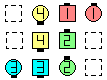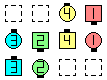\$B\$NA0(B \$B8e(B

In the above example, the two 8-spot formations are: EN: 50
\$B>e\$NNc\$G(B, 2 \$B\$D\$N(B 8-spot formation \$B\$O(B: JP: 50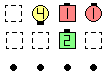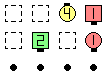upper 2 x 4 EN: 60 \$B>e\$N(B 2 x 4 JP: 60 before upper 2 x 4 EN: 60 \$B>e\$N(B 2 x 4 JP: 60 after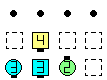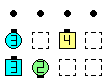lower 2 x 4 EN: 70 \$B2<\$N(B 2 x 4 JP: 70 before lower 2 x 4 EN: 70 \$B2<\$N(B 2 x 4 JP: 70 after

Triple Lines Working Backward Vertical Tag The Line: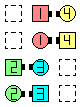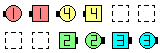\$B\$NA0(B \$B8e(B

In the above example, the two 8-spot formations are: EN: 50
\$B>e\$NNc\$G(B, 2 \$B\$D\$N(B 8-spot formation \$B\$O(B: JP: 50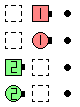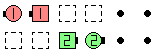leftmost 2 x 4 EN: 100 \$B:8\$N(B 2 x 4 JP: 100 before leftmost 2 x 4 EN: 100 \$B:8\$N(B 2 x 4 JP: 100 after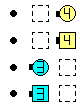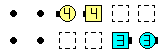rightmost 2 x 4 EN: 120 \$B1&\$N(B 2 x 4 JP: 120 before rightmost 2 x 4 EN: 120 \$B1&\$N(B 2 x 4 JP: 120 after

Triple Boxes Working Left Regroup: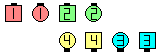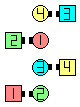Triple BoxesWorking Left Regroup\$B\$NA0(B \$B8e(B